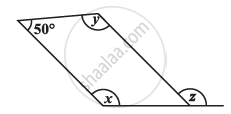# Consider the Given Parallelograms. Find the Values of the Unknowns X, Y, Z. -1 - Mathematics

Consider the given parallelograms. Find the values of the unknowns x, y, z.#### Solution

50° + y = 180° (Adjacent angles are supplementary)

y = 130°

x = y = 130° (Opposite angles are equal)

z = x = 130º (Corresponding angles)

Concept: Types of Quadrilaterals - Properties of a Parallelogram
Is there an error in this question or solution?

#### APPEARS IN

NCERT Class 8 Maths